# Some multiple Dirichlet series of completely multiplicative arithmetic functions

Nabil Tahmi and Abdallah Derbal
Notes on Number Theory and Discrete Mathematics
Print ISSN 1310–5132, Online ISSN 2367–8275
Volume 28, 2022, Number 4, Pages 603–616
DOI: 10.7546/nntdm.2022.28.4.603-616

## Details

### Authors and affiliations

Nabil TahmiDepartment of Mathematics, ENS of Laghouat and EDPNLHM Laboratory,
ENS of Kouba, Algiers, Algeria

Abdallah DerbalDepartment of Mathematics, EDPNLHM Laboratory,
ENS of Kouba, Algiers, Algeria

### Abstract

Let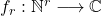be an arithmetic function of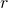variables, where. We study multiple Dirichlet series defined by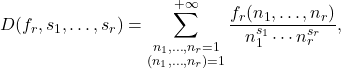where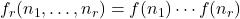and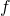is a completely multiplicative or a specially multiplicative arithmetic function of a single variable. We obtain formulas for these series expressed by infinite products over the primes. We also consider the cases of certain particular completely multiplicative and specially multiplicative functions.

### Keywords

• Completely multiplicative function
• Specially multiplicative function
• Multiple Dirichlet series
• Eulerian product
• Riemann zeta function
• Dirichlet L-function

### 2020 Mathematics Subject Classification

• 11M32, 11M06, 11A25

### References

1. Apostol, T. M. (1976). Introduction to Analytic Number Theory, Springer-Verlag, New York.
2. McCarthy, P. J. (1986). Introduction to Arithmetical Functions. Springer.
3. Nowak, W. G., & Tóth, L. (2014). On the average number of subgroups of the group Zm × Zn. International Journal of Number Theory, 10(2), 363–374.
4. Tóth, L. (2010). A survey of gcd-sum functions. Journal of Integer Sequences, 13, 2–3. (Article 10.8.1).
5. Tóth, L. (2011). Menon’s identity and arithmetical sums representing functions of several variables. Rendiconti del Seminario Matematico Universita e Politecnico di Torino, 69, 97–110.
6. Tóth, L. (2013). Two generalizations of the Busche–Ramanujan identities.  International Journal of Number Theory, 9, 1301–1311.
7. Tóth, L. (2014). Multiplicative Arithmetic Functions of Several Variables: A Survey. In: Rassias, Th. M., & Pardalos, P. (Eds.) Mathematics Without Boundaries, Surveys in Pure Mathematics. Springer, New York, pp. 483–514.
8. Tóth, L., & Zhai, W. (2010). On multivariable averages of divisor functions. Journal of Number Theory, 192(1), 251–269.